The ADDRESS function in Excel creates a cell reference as text, based on a given row and column number.

1. The ADDRESS function below returns \$E\$8. The row number is 8 and the column number is 5.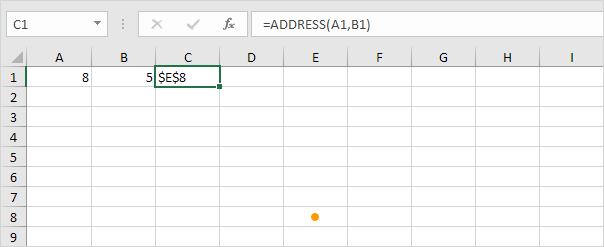2. The ADDRESS function below returns \$G\$3. The row number is 3 and the column number is 7.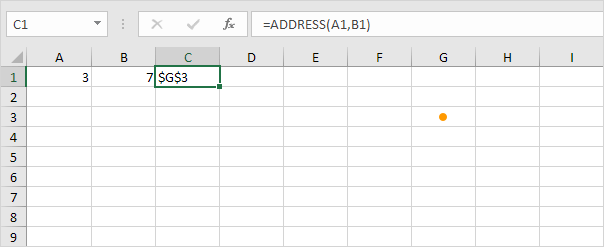3. The ADDRESS function returns a text value. Use the INDIRECT function in Excel to convert this text value into a valid cell reference.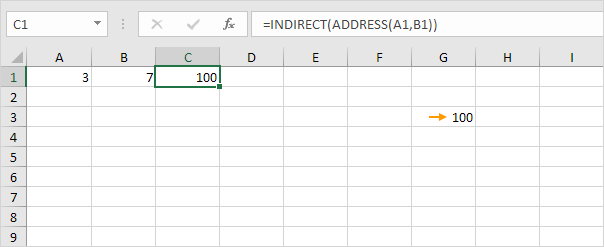4. By default, the ADDRESS function creates an absolute reference. To create a relative reference, use 4 for the third argument.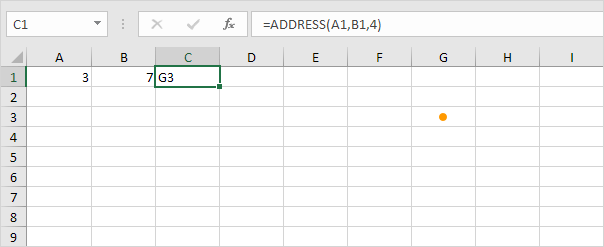5. For example, use the ADDRESS function in Excel to find the cell address of the maximum value in a column.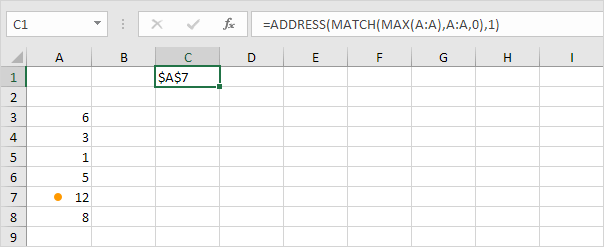Note: visit our page about locating the maximum value in a column for detailed instructions on how to create this formula.

6. Simply use the INDEX function in Excel to return a specific value in a two-dimensional range.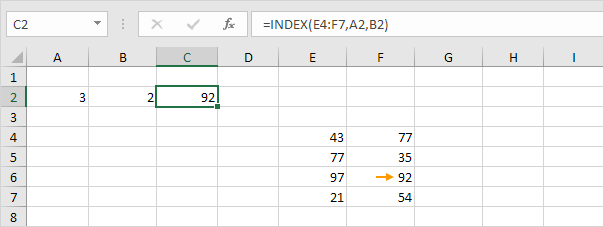Explanation: 92 found at the intersection of row 3 and column 2 in the range E4:F7. Use INDEX and MACTH in Excel to perform advanced lookups.